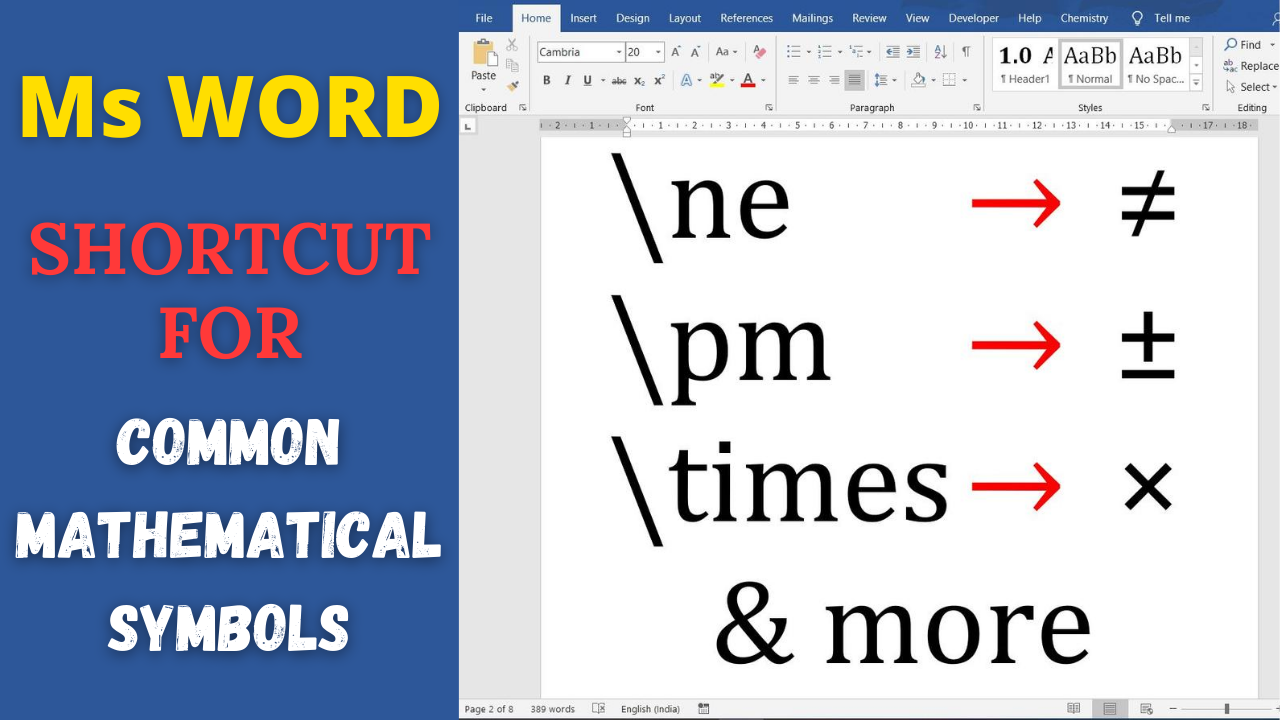# LaTeX in Word: Shortcut of typing degree symbol, different types of arrows and other mathematical symbolsMaths, Science and Engineering uses many unique symbols for denoting specific things. It includes symbols like infinity, different arrows, set symbols, degree sign, angle sign and various mathematical symbols and signs.

## Maths Symbol in Ms Word

Ms Word provides different ways to insert symbols. To add any symbol, you can go to Insert Tab and click on Symbols. This opens up the dialogue box, where you can find all different symbols for use. However, this method is time consuming when you need to use them often. You can type math equation and symbols really faster if you use Math Autocorrect shortcut of Ms Word.

## LaTeX in Word: Math Autocorrect

Math autocorrect provides LaTeX type facility to Ms Word for typing equation. These shortcuts are like LaTeX shortcuts and works inside equation editor (use Alt + = to get equation editor).

To enable Math Autocorrect outside equation editor, you need to do one time setting.

• Navigate Files > Options > Proofing > AutoCorrect Options and
• From Math AutoCorrect tab, check box which says “Use Math AutoCorrect rules outside math region”.

## Equation editor shortcut of degree symbols, different arrows and other mathematical symbols

Following table present equation editor shortcut for infinite, hbar, all kinds of arrows (for typing above/below arrow visit here), partial symbol, nabla, less than equal, greater than equal, times, oplus, ominus, mapsto, arrow with hook, congruent, approximately sign, therefore sign, because sign, for all sign, plus minus, minus plus, angle, proto, degree and many more.

## Conclusion

Equation editor shortcut provides a quick way to write Scientific and Mathematical symbols in Ms Word. Using them you can quickly type variety of arrows, set symbols, partial, nabla, degree C, angle, etc.

## Request

Hope that above shortcut helps you to type mathematical equation in Ms Word faster. If you find some symbols that are missing, comment, we will try to add it as soon as possible.

## 7 thoughts on “LaTeX in Word: Shortcut of typing degree symbol, different types of arrows and other mathematical symbols”

1.I cannot thank you enough for the article. Much thanks again. Cool!.

2.Hi, I think your blog might be having browser compatibility issues. When I look at your blog in Firefox, it looks fine but when opening in Internet Explorer, it has some overlapping. I just wanted to give you a quick heads up! Other then that, fantastic blog!

1.How to type A union B

1.To type A union B, type A \bigcup B, \bigcpu turns to union symbol.

3.Very helpful shortcut, Thanks for sharing!

4.This is much needed and very appreciated. Thank you.

1.Welcome dear. Thanks for writing your feedback. 🙂